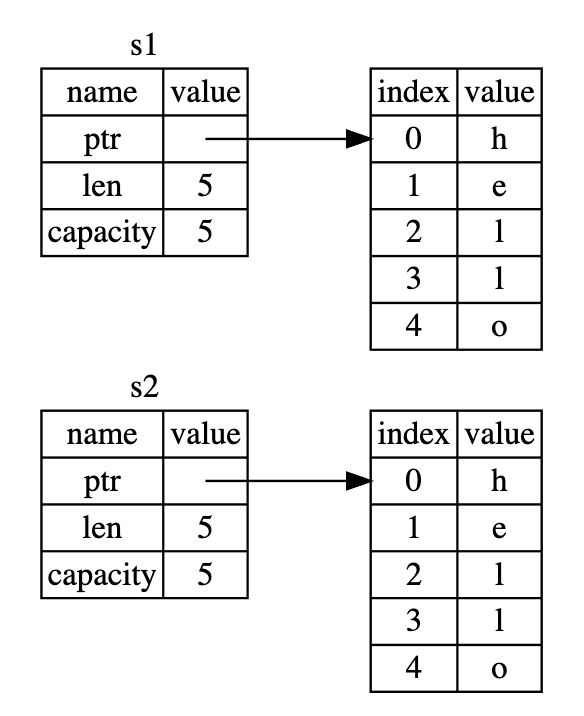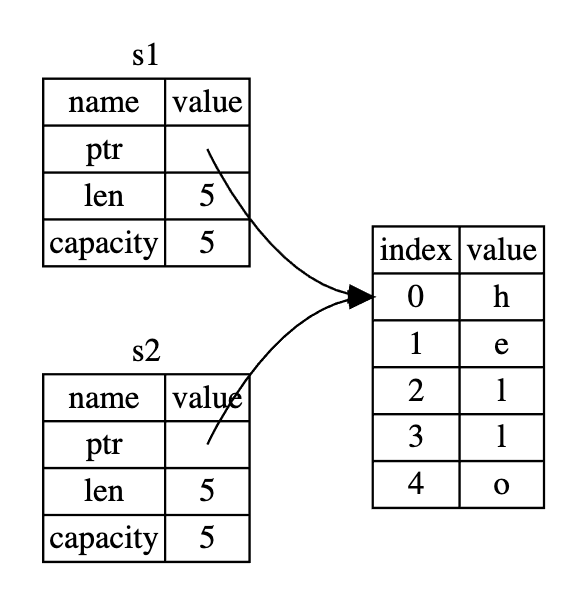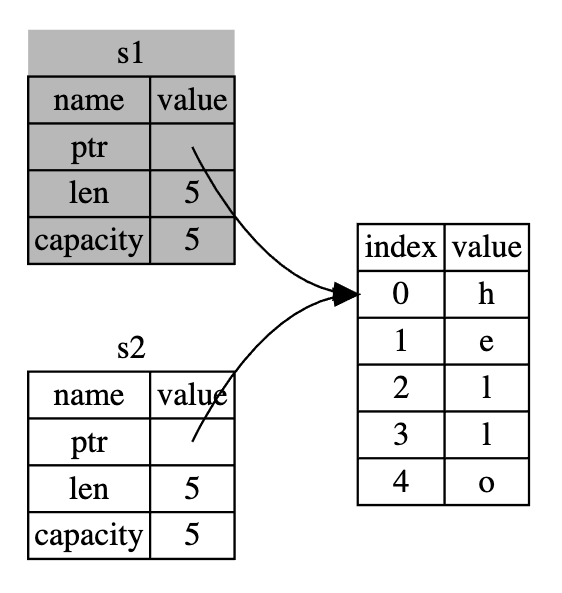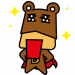#DAY 13
0

## Ownership Rules

Each value in Rust has a variable that’s called its owner.
There can only be one owner at a time.
When the owner goes out of scope, the value will be dropped.

• 在 rust 中每個值都有一個叫做 owner 的變數
• owner 一次只能有一個
• 如果 owner 離開 scope 他就會把他的值丟掉

## Variable Scope

``````fn main() {
let s = "hello";
}
// 在外面就無法存取 s 這個變數了
``````

## The `String` Type

``````let mut guess = String::new();
``````

``````let mut guess = "";
``````

## Memory and Allocation

``````let mut guess = String::new();

``````

• 這個變數必須在 runtime (執行期間)才能得到他的值
• 在完成 String 之後我們需要他返回一個一個 pointer 來讓我們操作

``````{
let s = String::from("hello");
}
``````

## Move vs Copy

``````fn move_vs_copy() {
// 這邊的 y 在記憶體中複製了一整份完整的資料
let x = 5;
let y = x;
println!("{}", x);
// s1 的指標和長度等資訊轉移給 s2
let s1 = String::from("hello");
let s2 = s1;
println!("{}", s1);
^^ value borrowed here after move
}
``````

### Copy• All the integer types, such as u32.
• The Boolean type, bool, with values true and false.
• All the floating point types, such as f64.
• The character type, char.
• Tuples, if they only contain types that are also Copy. For example, (i32, i32) is Copy, but (i32, String) is not.

### Move(反灰代表不能使用)

## Clone

``````let s1 = String::from("hello");
let s2 = s1.clone();

println!("s1 = {}, s2 = {}", s1, s2);
``````

## 最後一樣有問題歡迎發問## 參考網站

ch04-01-what-is-ownership